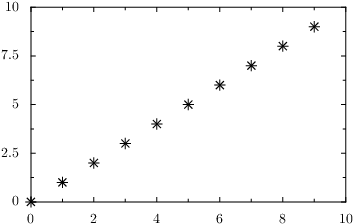# PyX — Example: graphs/points.py

0.4 KB
33.5 KB
37.4 KB
18.4 KB
20.1 KB

## Plotting data contained in one or more lists```# suggested by Chris Spencer

from pyx import *

g = graph.graphxy(width=8)
# either provide lists of the individual coordinates
g.plot(graph.data.values(x=list(range(10)), y=list(range(10))))
# or provide one list containing the whole points
g.plot(graph.data.points(list(zip(range(10), range(10))), x=1, y=2))
g.writeEPSfile("points")
g.writePDFfile("points")
g.writeSVGfile("points")
```

### Description

Here, we explain how to plot data which has been calculated before and which is available in a list.

The procedure is quite the same as for plotting a data file or a function in the above examples. It differs in that we use an instance of `graph.data.values` or `graph.data.points` in the `plot` method of the graph. The choice of one or the other variant depends on convenience and how the data has been generated: In `graph.data.values` the coordinate values are provided as individual lists. Alternatively, `graph.data.points` takes a list of data points which come as coordinate tuples. You will then have to say something like `x=1, y=2` to specify which entry in your tuples is to be used on which axis.

Similarly to the plotting of a data file, the default plotting style here are symbols.

Caution: This feature has been renamed/introduced between versions 0.9 and 0.10. In versions 0.9 and before the functionality of `graph.data.points` was provided by `graph.data.list`.